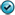## Exercises - Riemann Sums

1. Find the area under the curve $y = x^2$ from $x=2$ to $x=5$ by evaluating a limit of a Riemann sum.2. Find the area bound by the curve $y = x^3 - 5$ and the $x$-axis between $x=0$ and $x=2$ by evaluating a limit of a Riemann sum.3. The force required to stretch a spring $x$ units beyond its natural length of 14 cm is found to be $F(x) = 25x$. Setup and evaluate the limits of three different Riemann sums to determine how much work is done in stretching the spring from its natural length to a length of 18 cm. In the first, choose, as the representative $x$ value for the $i^{th}$ sub-interval, the left endpoint of that interval. In the second, choose the midpoint of each such interval, instead. In the third, choose the right endpoint.